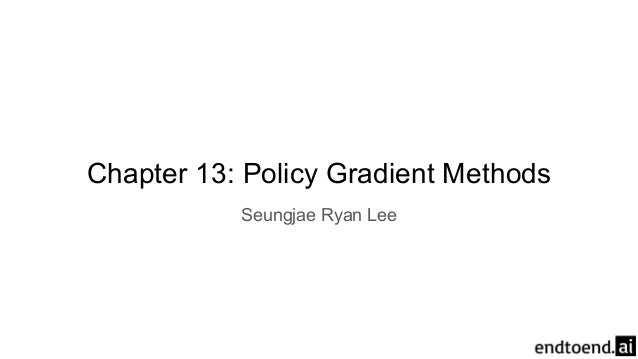Successfully reported this slideshow.
We use your LinkedIn profile and activity data to personalize ads and to show you more relevant ads. You can change your ad preferences anytime.Upcoming SlideShare
×

Reinforcement Learning 13. Policy Gradient Methods

267 views

Published on

A summary of Chapter 6: Policy Gradient Methods of the book 'Reinforcement Learning: An Introduction' by Sutton and Barto. You can find the full book in Professor Sutton's website: http://incompleteideas.net/book/the-book-2nd.html

Check my website for more slides of books and papers!
https://www.endtoend.ai

Published in: Technology
• Full Name
Comment goes here.

Are you sure you want to Yes No
Your message goes here• Be the first to comment

• Be the first to like this

Reinforcement Learning 13. Policy Gradient Methods

1. 1. Chapter 13: Policy Gradient Methods Seungjae Ryan Lee
2. 2. Preview: Policy Gradients ● Action-value Methods ○ Learn values of actions and select actions with estimated action values ○ Policy derived from action-value estimates ● Policy Gradient Methods ○ Learn parameterized policy that can select action without a value function ○ Can still use value function to learn the policy parameter
3. 3. Policy Gradient Methods ● Define a performance measure J(θ) to maximize ● Learn policy parameter θ through approximate gradient ascent Stochastic estimate of J(θ)
4. 4. Soft-max in Action Preferences ● Numerical preference h(s, a, θ) for each state-action pair ● Action selection through soft-max
5. 5. Soft-max in Action Preferences: Advantages 1. Approximate policy can approach deterministic policy ○ No “limit” like ε-greedy methods ○ Using soft-max on action values cannot approach deterministic policy
6. 6. Soft-max in Action Preferences: Advantages 2. Allow stochastic policy ○ Best approximate policy can be stochastic in problems with significant function approximation ● Consider small corridor with -1 reward on each step ○ States are indistinguishable ○ Action transition is reversed in the second state
7. 7. Soft-max in Action Preferences: Advantages 2. Allow stochastic policy ○ ε-greedy methods (ε=0.1) cannot find optimal policy
8. 8. Soft-max in Action Preferences: Advantages 3. Policy may be simpler to approximate ○ Differs among problems http://proceedings.mlr.press/v48/simsek16.pdf
9. 9. Theoretical Advantage of Policy Gradient Methods ● Smooth transition of policy for parameter changes ● Allows for stronger convergence guarantees h = 1 h = 0.9 0.4750.525 h = 1 h = 1.1 0.475 0.525 Q = 1 Q = 0.9 0.050.95 Q = 1 Q = 1.1 0.5 0.95
10. 10. Policy Gradient Theorem ● Define performance measure as value of the start state ● Want to compute w.r.t. policy parameter
11. 11. Policy Gradient Theorem Episodic: Average episode length Continuing: 1 On-policy state distribution
12. 12. Policy Gradient Theorem: Proof
13. 13. Policy Gradient Theorem: Proof Product rule
14. 14. Policy Gradient Theorem: Proof
15. 15. Policy Gradient Theorem: Proof
16. 16. Policy Gradient Theorem: Proof Unrolling
17. 17. Policy Gradient Theorem: Proof
18. 18. Policy Gradient Theorem: Proof
19. 19. Policy Gradient Theorem: Proof
20. 20. Policy Gradient Theorem: Proof
21. 21. Policy Gradient Theorem: Proof
22. 22. Stochastic Gradient Descent ● Need samples with expectation
23. 23. Stochastic Gradient Descent is an on-policy state distribution of
24. 24. Stochastic Gradient Descent
25. 25. Stochastic Gradient Descent
26. 26. Stochastic Gradient Descent: REINFORCE
27. 27. REINFORCE (1992) ● Sample return like Monte Carlo ● Increment proportional to return ● Increment inverse proportional to action probability ○ Prevent frequent actions dominating due to frequent updates
28. 28. REINFORCE: Pseudocode Eligibility vector
29. 29. REINFORCE: Results
30. 30. REINFORCE with Baseline ● REINFORCE ○ Good theoretical convergence ○ Bad convergence speed due to high variance Baseline
31. 31. REINFORCE with Baseline: Pseudocode Learn state value with MC to use as baseline
32. 32. REINFORCE with Baseline: Results
33. 33. ● Learn approximations for both policy (Actor) and value function (Critic) ● Critic vs Baseline in REINFORCE ○ Critic is used for bootstrapping ○ Bootstrapping introduces bias and relies on state representation ○ Bootstrapping reduces variance and accelerates learning Actor-Critic Methods Actor Critic
34. 34. One-step Actor Critic ● Replace return with one-step return ● Replace baseline with approximated value function (Critic) ○ Learned with semi-gradient TD(0) REINFORCE: One-step AC:
35. 35. One-step Actor-Critic Update Critic (value function) parameters Update Actor (policy) parameters
36. 36. Actor-Critic with Eligibility Traces
37. 37. Average Reward for Continuing Problems ● Measure performance in terms of average reward
38. 38. Actor-Critic for Continuing Problems
39. 39. Policy Gradient Theorem Proof (Continuing Case) 1. Same procedure: 2. Rearrange equation:
40. 40. Policy Gradient Theorem Proof (Continuing Case) 3. Sum over all states weighted by state-distribution a. Nothing changes since neither side depend on s and
41. 41. Policy Gradient Theorem Proof (Continuing Case) 4. Use ergodicity:
42. 42. ● Policy based methods can handle continuous action spaces ● Learn statistics of the probability distribution ○ ex) mean and variance of Gaussian ● Choose action from the learned distribution ○ ex) Gaussian distribution Policy Parameterization for Continuous Actions
43. 43. Policy Parametrization to Gaussian Distribution ● Divide policy parameter vector into mean and variance: ● Approximate mean with linear function: ● Approximate variance with exponential of linear function: ○ Guaranteed positive ● All PG algorithms can be applied to the parameter vector
44. 44. Summary ● Policy Gradient methods have many advantages over action-value methods ○ Represent stochastic policy and approach deterministic policies ○ Learn appropriate levels of exploration ○ Handle continuous action spaces ○ Compute effect of policy parameter on performance with Policy Gradient Theorem ● Actor-Critic estimates value function for bootstrapping ○ Introduces bias but is often desirable due to lower variance ○ Similar to preferring TD over MC “Policy Gradient methods provide a significantly different set of strengths and weaknesses than action-value methods.”
45. 45. Thank you! Original content from ● Reinforcement Learning: An Introduction by Sutton and Barto You can find more content in ● github.com/seungjaeryanlee ● www.endtoend.ai